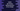# Kotlin program to get binary representation of integer## Introduction :

Kotlin program to get the binary representation of an integer value. We have two different ways to find out the binary representation of an integer value. Let me show you how :

### toBinaryString :

This method is defined in Integer class. It is defined as below :

``public static String toBinaryString(int i)``

It returns the binary representation of an integer. Its return value is of type string.

For example :

``````fun main() {
println(Integer.toBinaryString(10))
println(Integer.toBinaryString(4))
println(Integer.toBinaryString(101212))
}``````

It will print :

``````1010
100
11000101101011100``````

### Using toString :

toString method of Kotlin integer class optionally takes one integer radix value. If it is 2, it returns the binary representation of that integer. For example :

``````fun main() {
println(10.toString(2))
println(4.toString(2))
println(101212.toString(2))
}``````

It will print the same output as the above example.